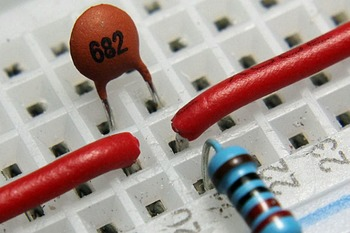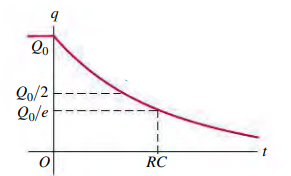R-C Circuit of Direct CurrentIn this circuit, Resistors and Capacitors are main components along with an emf source. This type of circuit in which a capacitor is alternately charged  and discharged is widely incorporated by many electronic devices such as electronic flash units, vehicles turn signals and traffic signals.  In this process of charging and discharging capacitor, the currents, voltages, and powers are dependent with time.fig: R-C circuit

In the above circuit, when the switch S is closed on a, then the charging of capacitor occurs and when closed on b discharging occurs.

Charging Capacitor

In time dt a charge dq moves through the circuit and deposited on positive plate of the capacitor. The work done by the source of emf must equal the internal energy produced in the resistor during time dt, plus the increase dU in the amount of energy U that is stored in the capacitor. Conservation of energy gives,dividing by dt gives,The result is analogous to that of Loop rule as this rule is derived from conservation of energy principle.

Arranging and integrating the above equation,Solving for q, we get,................(i)fig: above graphs shows accumulation of charge on capacitor and decrement of current in             resistor .

The term RC in the above equations has dimension of time as the power of exponential should be dimensionless. It is called capacitive time constant.When we input t=RC then we obtain,So, It is the time at which the charge on the capacitor has increased to within a factor of 1 — e-1 (~ 63%) of its final value.

Discharging Capacitor

After the capacitor is fully charged and no current is flowing, the switch in the circuit is closed on b. Now, the capacitor is discharged through the resistor. As there is no emf, our equation simply becomes,Rearranging and integrating, we getsolving for q we get,where q0 is initial charge which is given by,Then,The negative sign denotes the capacitor is discharging rather charging.fig: Graphs showing decrease in charge and current for discharging capacitor.